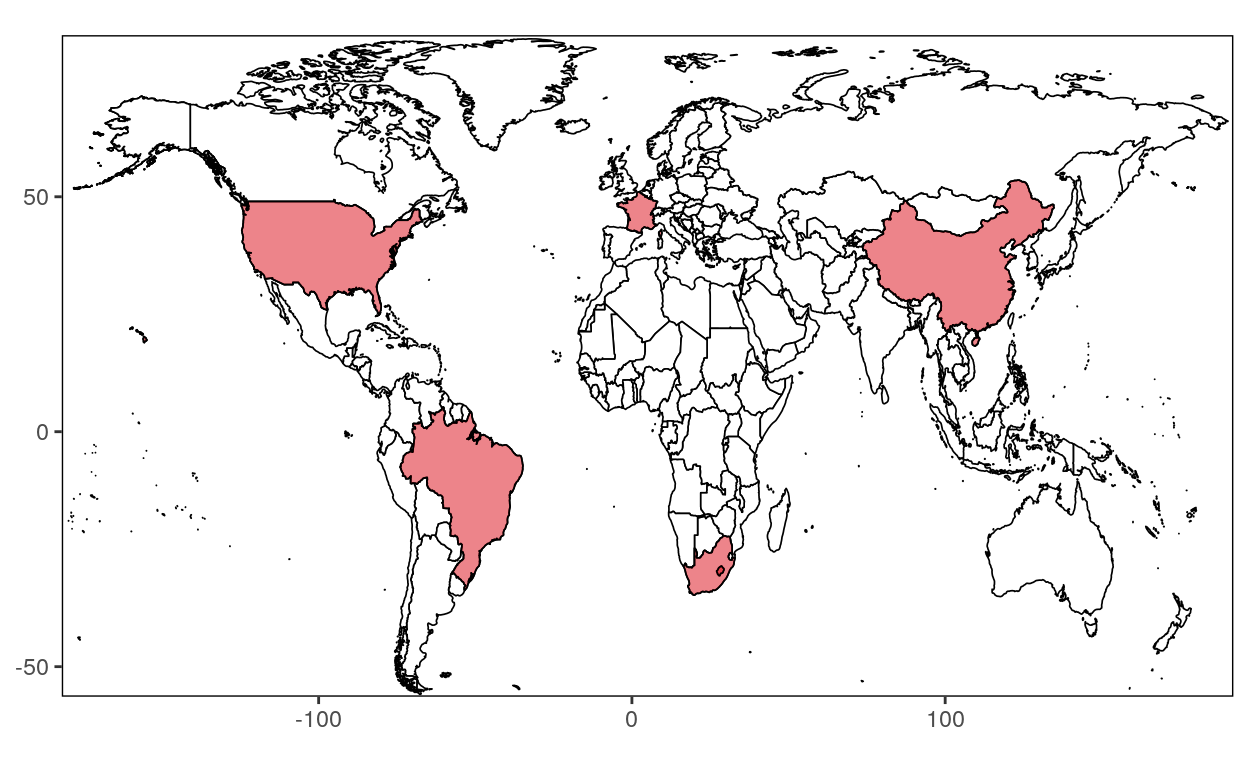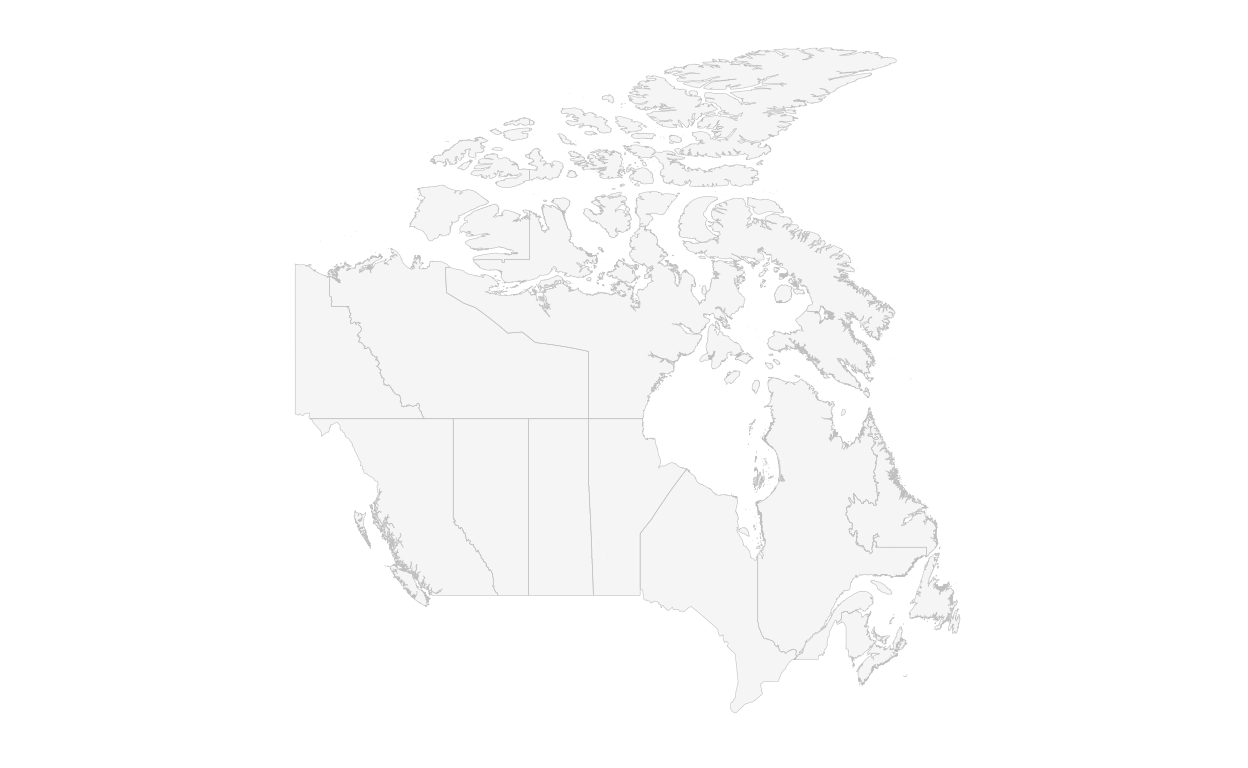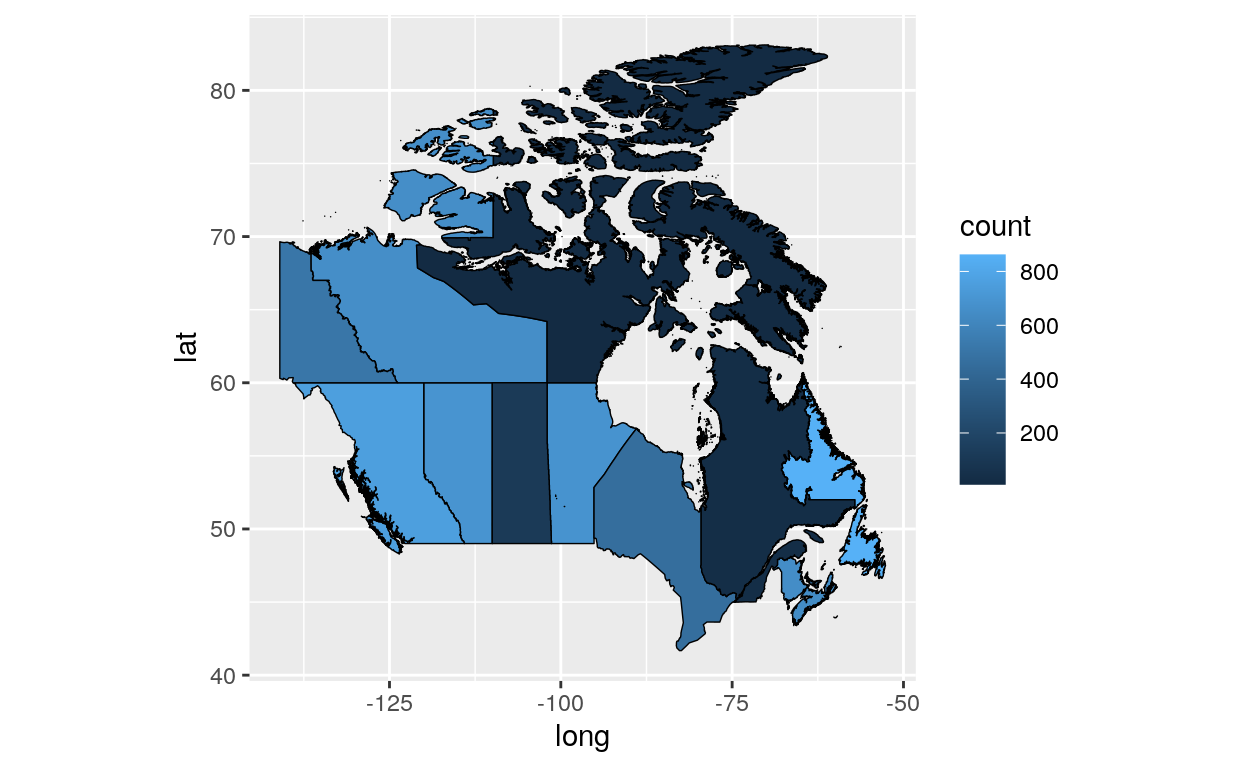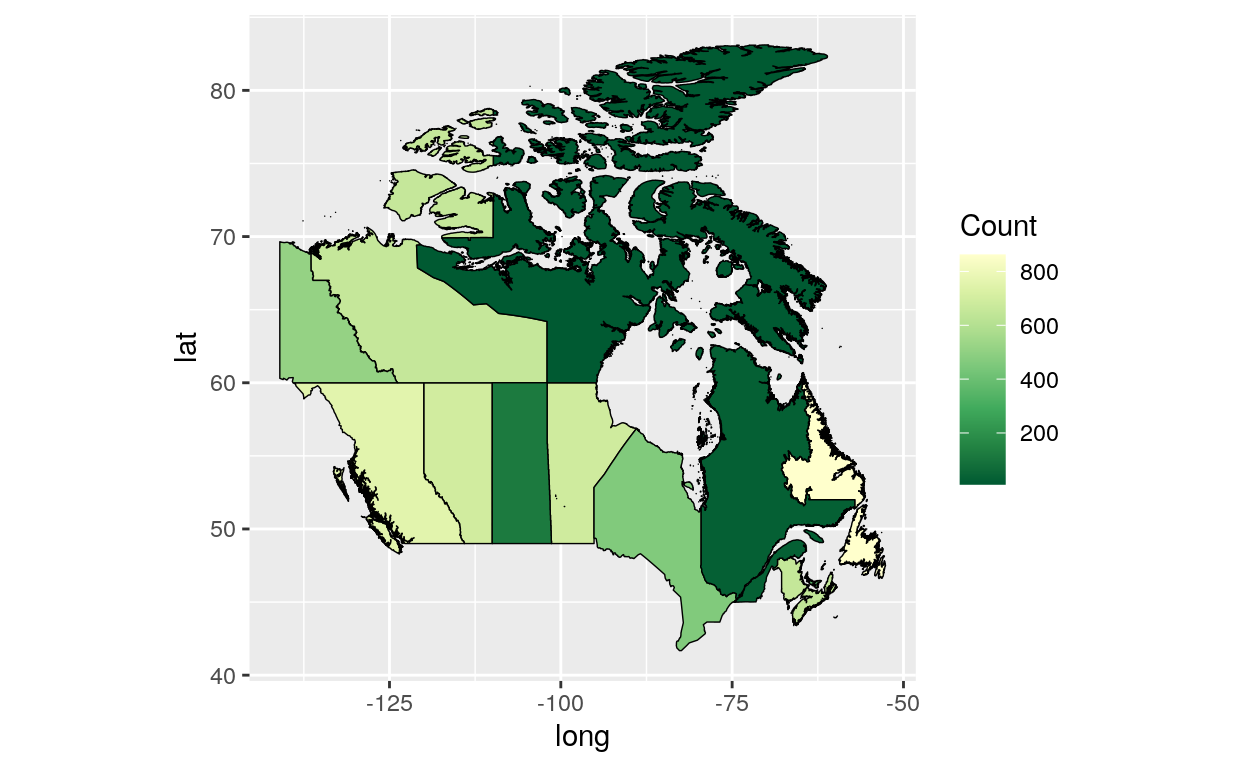# [R Course] Data Visualization with R: Colored Map Levels

A map with colored levels of granularity made using the raster and ggplot2 packages.

01-30-2020This course will teach you how to draw lines to divide a country into smaller regions and add colors. This tutorial is an extension of the following course: Data Visualization with R: Map Levels.

``````library(maptools)
library(raster)
library(plyr)
library(ggplot2)
library(rgdal)
``````

## Retrieve and manipulate data

``````Canada1 <- getData('GADM', country="CAN", level=1)

``````
``````  "Alberta"                   "British Columbia"
 "Manitoba"                  "New Brunswick"
 "Newfoundland and Labrador" "Northwest Territories"
 "Nova Scotia"               "Nunavut"
 "Ontario"                   "Prince Edward Island"
 "Yukon"                    ``````
``````Quebec<-Canada1[Canada1@data\$NAME_1 == "Québec",]
Quebec_df<-fortify(Quebec)

count<-sample(1:1000,13)     #or any other data you can associate with admin level here

count_df<-data.frame(NAME_1, count)

``````

## Create map

``````theme_opts<-list(theme(panel.grid.minor = element_blank(),
panel.grid.major = element_blank(),
panel.background = element_blank(),
plot.background = element_blank(),
axis.line = element_blank(),
axis.text.x = element_blank(),
axis.text.y = element_blank(),
axis.ticks = element_blank(),
axis.title.x = element_blank(),
axis.title.y = element_blank(),
plot.title = element_blank()))

ggplot() +
geom_path(data=Canada1_df, aes(long,lat, group=group), color="grey", size=0.1) +
theme(aspect.ratio=1)+
theme_opts
````````````ggplot() +
geom_polygon(data = Canada1_df, aes(x = long, y = lat, group = group, fill =
count), color = "black", size = 0.25) +
theme(aspect.ratio=1)
````````````library(scales)
ggplot() +
geom_polygon(data = Canada1_df, aes(x = long, y = lat, group = group, fill =
count), color = "black", size = 0.25) +
theme(aspect.ratio=1)+
scale_fill_distiller(name="Count", palette = "YlGn", breaks = pretty_breaks(n = 5))
``````### Citation

`Warin (2020, Jan. 30). Thierry Warin, PhD: [R Course] Data Visualization with R: Colored Map Levels. Retrieved from https://warin.ca/posts/rcourse-datavisualizationwithr-coloredmapslevels/`
```@misc{warin2020[r,